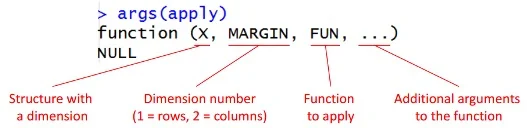# R : Apply Function on Rows

This tutorial explains how to apply functions on rows.

Sample Data
X Y Z
6 5 0
6 3 NA
6 1 5
8 5 3
1 NA 1
8 7 2

Apply Function

When we want to apply a function to the rows or columns of a matrix or data frame. It cannot be applied on lists or vectors.apply arguments

Calculate maximum value across row
apply(data, 1, max)
It returns NA if NAs exist in a row. To ignore NAs, you can use the following line of code.
apply(data, 1, max, na.rm = TRUE)
Calculate mean value across row
apply(data, 1, mean)
apply(data, 1, mean, na.rm = TRUE)
Calculate number of 0s in each row
apply(data == 0, 1, sum, na.rm= TRUE)
Calculate number of values greater than 5 in each row
apply(data > 5, 1,  sum, na.rm= TRUE)
Select all rows having mean value greater than or equal to 4
df = data[apply(data, 1, mean, na.rm = TRUE)>=4,]
Remove rows having NAs
helper = apply(data, 1, function(x){any(is.na(x))})
df2 = data[!helper,]
It can be easily done with df2 = na.omit(data).

Count unique values across row
df3 = apply(data,1, function(x) length(unique(na.omit(x))))
Related Posts
Share1.